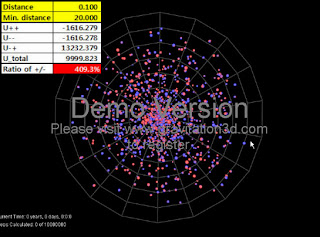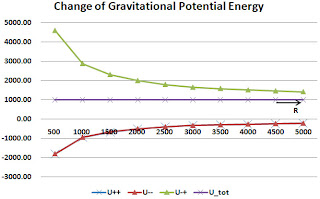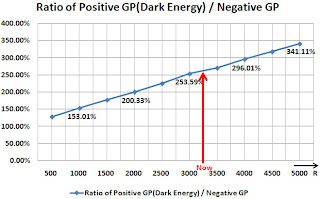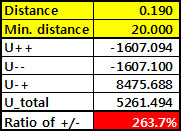Gravity is the force conquering the structure of the universe. By recognizing the components of the universe, we are estimating the quantity of components composing the universe through size of gravity and gravitational potential energy (GPE).

In this paper, it is being shown that the universe can be created and expanded through pair creation of positive energy (mass) and negative energy (mass) from zero energy condition.

Also, GPE is composed by 3 units of U++, U--, and U-+ when negative and positive energy exists, U-+ (GPE between negative mass and positive mass) has positive values and is the component that makes repulsive gravitational effect. U-+ corresponds with the inner energy of the system and can be interpreted as dark energy.

Also, situations in which U-+ has much higher value than |U-- + U++| depending on the distribution of negative mass and positive mass is possible.

This doesn't mean that 72.1% of dark energy independently exists, but means that explanation from GPE occurring from 4.6%(or about 27.9%) of negative energy, which is the same as 4.6%(or include dark matter 27.9%) of positive energy, is possible. Moreover, 4.6%(or 27.9%) of negative energy is the energy which is inevitably required from zero energy, which is the most natural total energy value in the universe.

This discovery implies that our belief that size of gravity and size of components of the universe would always 1:1 correspond was wrong.

** Simulation Program
To look into the characteristic of GPE, we used the simulation program named Gravitation3D made by Roice Nelson.

**Simulation setting
a)Definition of parameter
A few parameters were needed to be defined for simulation. Distance between pair creation negative energy and positive energy(distance of 1 pair) : d0
Minimum distance between particle pairs for density modification during pair creation : dm
Radius of pair creation range : R0 = 500
Particle number of pair creation : N0 =2000ea (1000 pair)

b)Finding mean value
Through Gravitation3D program, 1000 particle pairs (total 2000ea particles) were produced by random and one mean value (GPE) of each distance value was found 5 times each.

c)Verification on program
To check if the calculated results of the program were correct, we calculated the GPE when 1, 2, and 3 pairs (consist of 15ea potentials) of particles existed by hand and confirmed that this value corresponded to the calculated results of the program.

B. Results of simulation

1) Distance=0.01It was found out that U-+ value having positive value could be much higher than |(U--) + (U++)|. Thus, even though the size of positive mass and negative mass was equal, it could be known that repulsive GPE could be much higher than attractive GPE.

2) Distance =0.10We will describe that an unknown repulsive energy U-+ higher that attraction of visible matter exists for the energy value of above.

......

C. Accelerating expansion of the universe

Expansion of the universe means increase of the distance between cluster of galaxies or the galaxy while the sizes of individual galaxies are the same.
Structure of galaxies or cluster of galaxies surrounding negative masses in the pair creation model of negative and positive mass is implied.

In this simulation, d0, which is the distance between negative and positive mass, is maintained as a constant in this simulation, or the radius value of the size of the universe increases when d0 decreases.Pay attention on the change of negative GPE and positive GPE ratioAs R, which corresponds to the radius of the universe, increases, absolute values of GPE all decrease while the total GPE is mostly maintained.However, ratio of positive gravitational potential and negative gravitational potential increases as the universe expands and this is seen to mean that percentage of repulsive effect (Dark Energy) increases compared to the attractive effect of ordinary matter.

This "increase of dark energy" doesn't come from a newly generated space, but shows feature of GPE which is made by negative mass and positive mass.

...........
H.Results of simulation
1) Even though negative and positive mass have the same size, total GPE can have +, 0, - values depending on the placement of each.
2) U-+ value is higher as d0 is smaller and the total GPE also have a high positive value.
3) Looking into the numerical calculation of B)-1)-B)-4), it can be seen that positive gravitational potential value U-+ can have much higher values than negative gravitational potential values(138% - 3,201%). If we consider the distance of Planck length level, this value can have very high values enough to explain inflation.
From the observation result of WMAP, we know that dark energy value, which generates repulsion, is 2.58 times the total of matter and dark matter. Until now, it is predicted that dark matter produces attraction. Closely look into numbers B)-3).4) Because we judge components of the universe by gravity or GPE, it can be assumed that there is dark energy of 2.63 times or inner energy of 2.63 times regarding the situation of U-+ value having approximately 2.63 times U++ and U-- values in number B)-3). There are several situations satisfying 2.58 times.

5) The above results show that 72.1% of dark energy doesn't exist independently, but implies that there is possibility that negative energy having the same size of positive energy can emerge.

6)This "increase of dark energy" doesn't come from a newly generated space, but shows feature of GPE which is made by negative mass and positive mass.

No one has seen dark energy or dark matter. We must remember that we only recognize it through gravitational effect.

When we judge the components of the universe, we judge the components by gravitational effect rather than mass energy.

Therefore, when gravitational potential energy U-+ exists larger than gravitational potential energy U which is generated by materials, we will be confused to think that some mass energy bigger than the mass energy of materials exists.

===========
Paper :
Important Report on the Dark Energy and Gravitational Potential Energy
http://vixra.org/abs/1110.0019